Ms. Matlock

•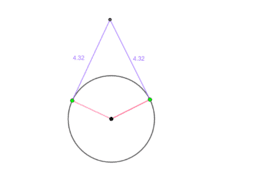Tangent Lines (P3)

Activity

Ms. Matlock

•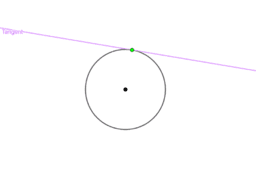Theorems with Tangents to Circles

Book

Ms. Matlock

•Proof with Tangent Line

Activity

Ms. Matlock

•Extension: 1

Activity

Ms. Matlock

•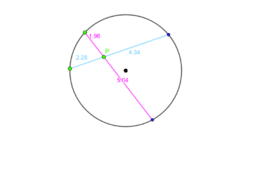Angles and Secants P2

Activity

Ms. Matlock

•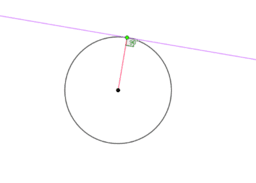Tangent Lines (P2)

Activity

Ms. Matlock

•Tangent Lines (P1)

Activity

Ms. Matlock

•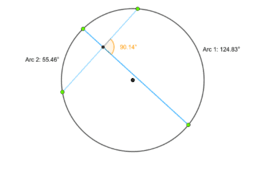Angles and Secants P3

Activity

Ms. Matlock

•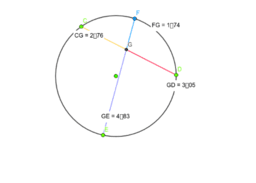Theorems with Secants and Angles

Book

Ms. Matlock

•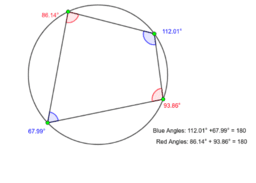Angles and Secants (P4)

Activity

Ms. Matlock

•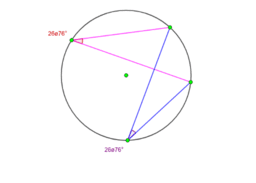Extension:

Activity

Ms. Matlock

•Angles and Secants P1.

Activity

Ms. Matlock

•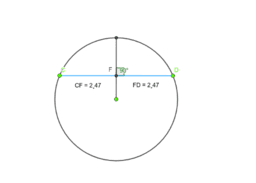Theorems with Chords of Circles

Book

Ms. Matlock

•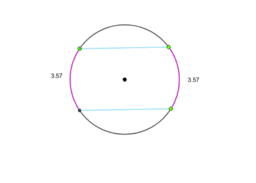Theorems with Chords P4

Activity

Ms. Matlock

•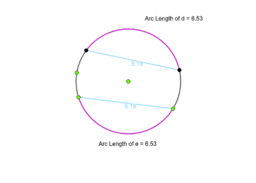Theorems with Chords P3

Activity

Ms. Matlock

•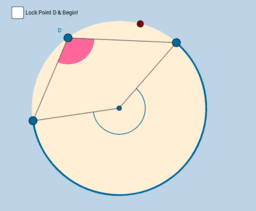Book

Ms. Matlock